### Home > PC > Chapter 4 > Lesson 4.1.2 > Problem4-30

4-30.
1. Given x2 + y2 = 1, simplify each of the following expressions. Homework Help ✎

1.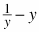2.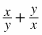3.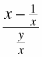4.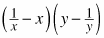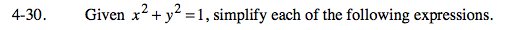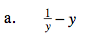Write with one common denominator.

$\frac{1-y^2}{y}$

Substitute y2 with 1 − x2 from the given equation.

$\frac{1-(1-x^2)}{y}$

Simplify.

$\frac{x^2}{y}$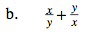Rewrite the expression with a common denominator.
Substitute from the given equation.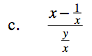Rewrite the expression in the numerator with a common denominator.
Simplify and substitute.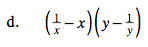1. Multiply out.
2. Make all fractions with the same common denominator.
3. Simplify.